# How to remove non numeric characters from a cell

This post explains that how to remove non-numeric characters (numbers) from a text string in one cell in excel 2016. How to remove non numeric characters from a cell containing text string with an excel formula. And how to remove alphanumeric characters from a cell with a user defined function in excel VBA.

## Remove non numeric characters with an Excel Formula

If you want to remove non numeric characters from a text cell in excel, you can use the array formula:

`{=TEXTJOIN("",TRUE,IFERROR(MID(B1,ROW(INDIRECT("1:"&LEN(B1))),1)+0,""))}`

Let’s see how the above formula works:

`=ROW(INDIRECT("1:"&LEN(B1))`

The ROW function returns the below array list:

`{1,2,3,4,5,6,7,8,9}`

`=MID(A1,ROW(INDIRECT("1:"&LEN(A1))),1)`

The MID formula will return the below array:

`{"e","x","c","e","l","2","0","1","6"}`

`=IFERROR(MID(B1,ROW(INDIRECT("1:"&LEN(B1))),1)+0,"")`

The array returned by the above MID function add zero for each value in array. If the value is a numeric text, it will be converted to text format. If not, returns empty string. So the IFERROR function returns the below array:

`{2,0,1,6}`

Last, the TEXTJOIN function join the values in above array returned by the IFERROR function.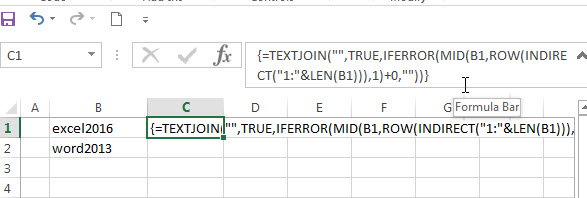## Remove non numeric characters using VBA function

You can create a new function to remove numeric characters from a cell that contain text string in Excel VBA. Just refer to the below steps:

#1 open visual Basic Editor, then insert a module and name as : RemoveNonNum.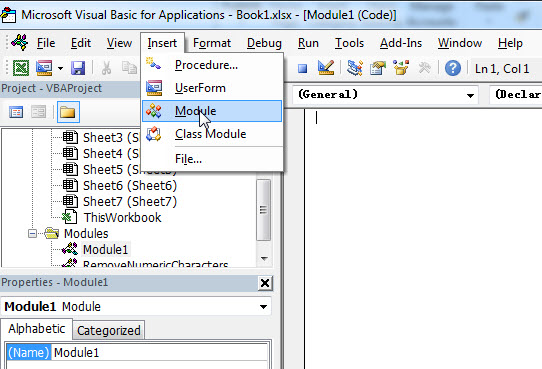#2 click “Insert“->”Module“, then paste the following VBA code into the window:

#3 paste the below VBA code into the code window. Then clicking “Save” button.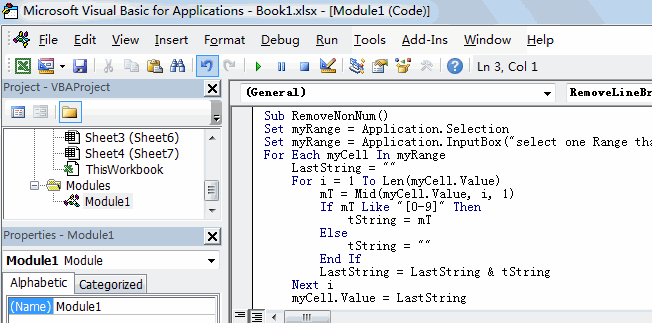```Sub RemoveNonNum()
Set myRange = Application.Selection
Set myRange = Application.InputBox("select one Range that you want to remove non numeric characters", "RemoveNonNum", myRange.Address, Type:=8)
For Each myCell In myRange
LastString = ""
For i = 1 To Len(myCell.Value)
mT = Mid(myCell.Value, i, 1)
If mT Like "[0-9]" Then
tString = mT
Else
tString = ""
End If
LastString = LastString & tString
Next i
myCell.Value = LastString
Next
End Sub```

#4 back to the current worksheet, then run the above excel macro. Click Run button.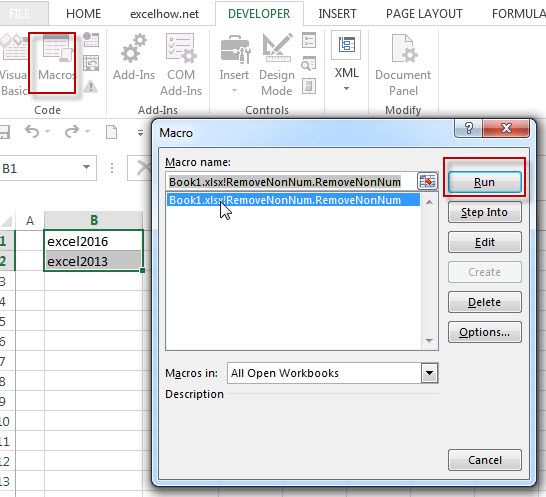#5 select one Range that you want to remove non numeric characters. click Ok button.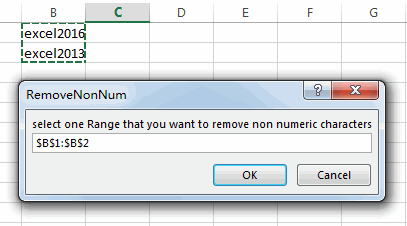#6 Let’s see the last result: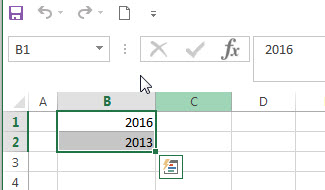### Related Formulas

• Remove Numeric Characters from a Cell
If you want to remove numeric characters from alphanumeric string, you can use the following complex array formula using a combination of the TEXTJOIN function, the MID function, the Row function, and the INDIRECT function..…
• Combine Text from Two or More Cells into One Cell
If you want to combine text from multiple cells into one cell and you can use the Ampersand (&) symbol.If you are using the excel 2016, then you can use a new function TEXTJOIN function to combine text from multiple cells…

### Related Functions

• Excel TEXTJOIN function
The Excel TEXTJOIN function joins two or more text strings together and separated by a delimiter. you can select an entire range of cell references to be combined in excel 2016.The syntax of the TEXTJOIN function is as below:= TEXTJOIN  (delimiter, ignore_empty,text1,[text2])…
• Excel MID function
The Excel MID function returns a substring from a text string at the position that you specify.The syntax of the MID function is as below:= MID (text, start_num, num_chars)…
• Excel LEN function
The Excel LEN function returns the length of a text string (the number of characters in a text string).The LEN function is a build-in function in Microsoft Excel and it is categorized as a Text Function.The syntax of the LEN function is as below:= LEN(text)…
• Excel IFERROR function
The Excel IFERROR function returns an alternate value you specify if a formula results in an error, or returns the result of the formula.The syntax of the IFERROR function is as below:= IFERROR (value, value_if_error)….
• Excel ROW function
The Excel ROW function returns the row number of a cell reference.The ROW function is a build-in function in Microsoft Excel and it is categorized as a Lookup and Reference Function.The syntax of the ROW function is as below:= ROW ([reference])….
• Excel INDIRECT  function
The Excel ROW function returns the row number of a cell reference.The ROW function is a build-in function in Microsoft Excel and it is categorized as a Lookup and Reference Function.The syntax of the ROW function is as below:= ROW ([reference])….

## Comments

### This post currently has 3 responses

•Mark Chapulis says:

I copied and pasted the formula, using C^S^E so that it became an array, and I changed the field of the desired cell from general to text, but when I changed the 2 cell locations in the formula to the location of the desired cell, I got “#NAME?”
What have I done wrong?

•Mark Chap says:

I copied and pasted the formula, using C^S^E so that it became an array, and I changed the field of the desired cell from general to text, but when I changed the 2 cell locations in the formula to the location of the desired cell, I got “#NAME?”
What have I done wrong?

•scott says:

Hi markchap68,

If you are using the excel 2013, it will return the #NAME!. As the TEXTJOIN function is only available in Excel 2016. So you can consider to use the VBA Macro to remove non numeric characters.

Sidebar